# The MDC Procedure

### Multinomial Probit

The multinomial probit model allows the random components of the utility of the different alternatives to be nonindependent and nonidentical. Thus, it does not have the IIA property. The increase in the flexibility of the error structure comes at the expense of introducing several additional parameters in the covariance matrix of the errors.

Consider the random utility function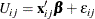where the joint distribution of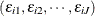is multivariate normal: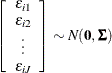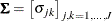The dimension of the error covariance matrix is determined by the number of alternatives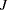. Given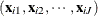, the jth alternative is chosen if and only if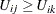for all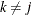. Thus, the probability that the jth alternative is chosen is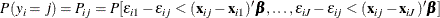whereis a random variable that indicates the choice made. This is a cumulative probability from a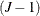-variate normal distribution. Since evaluation of this probability involves multidimensional integration, it is practical to use a simulation method to estimate the model. Many studies have shown that the simulators proposed by the following authors (henceforth referred to as GHK) perform well: Geweke (1989); Hajivassiliou (1993); Keane (1994). For example, Hajivassiliou, McFadden, and Ruud (1996) compare 13 simulators using 11 different simulation methods and conclude that the GHK simulation method is the most reliable. To compute the probability of the multivariate normal distribution, the recursive simulation method is used. Refer to Hajivassiliou (1993) for more details about GHK simulators.

The log-likelihood function for the multinomial probit model can be written as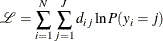where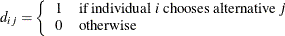For identification of the multinomial probit model, two of the diagonal elements of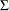are normalized to 1, and it is assumed that for one of the choices whose error variance is normalized to 1 (say, k), it is also true that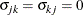for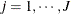and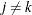. Thus, a model withalternatives has at most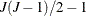covariance parameters after normalization.

Let
and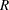be defined as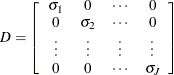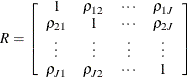where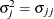and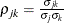. Then, for identification,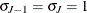and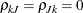, for all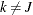can be imposed, and the error covariance matrix is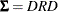.

In the standard MDC output, the parameter estimates STD_j and RHO_jk correspond to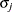and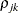.

In principle, the multinomial probit model is fully identified with the preceding normalizations. However, in practice, convergence in applications of the model with more than three alternatives often requires additional restrictions on the elements of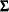.

It must also be noted that the unrestricted structure of the error covariance matrix makes it impossible to forecast demand for a new alternative without knowledge of the new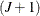byerror covariance matrix.